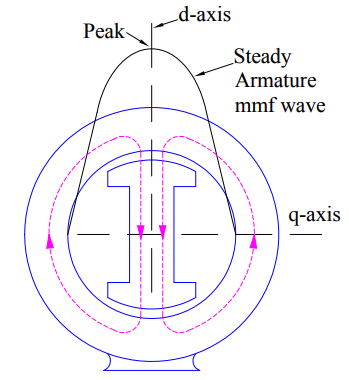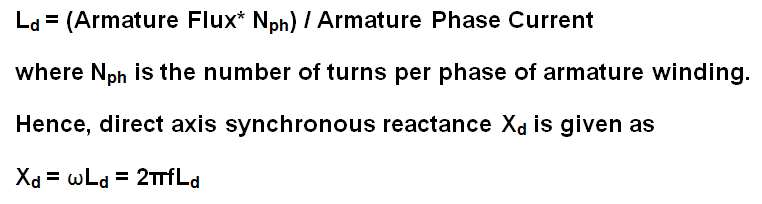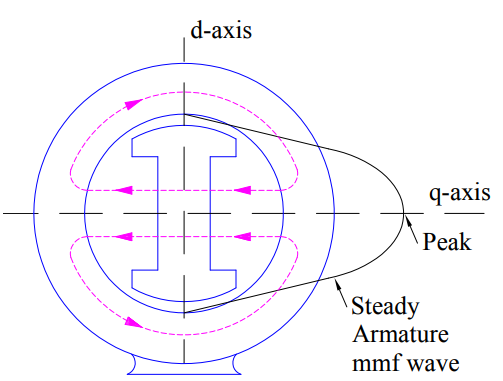# Understanding of Direct and Quadrature Axis Synchronous Reactance

### Direct Axis Synchronous Reactance

A direct axis quantity is one whose magnetic effect is along the field pole axis. Field pole axis is also known as direct axis. Based on this fact, a direct axis synchronous reactance is defined as the reactance offered to the armature flux when the peak of armature mmf is directed along the direct axis. Let us understand this more clearly.

In the figure below, it can easily be seen that the peak of armature mmf is directed along the field pole axis or direct axis.Under this condition, the air gap length is minimum and hence the reluctance is also minimum. Thus the armature flux (Flux = mmf / Reluctance) is maximum in this case. Under this condition, the flux linkage per ampere in the armature winding is called direct axis synchronous inductance Ld.

Thus when the peak of armature mmf wave coincides with the direct axis (often called d-axis), then the d-axis synchronous inductance Ld is given asA quadrature axis is one whose magnetic effect is along the perpendicular to field pole axis. When the peak of armature mmf coincides with the quadrature axis, the reactance offered to the armature mmf is called Quadrature Axis Reactance.In the above figure, the peak of armature mmf is along the perpendicular to the field pole axis or direct axis.Under this condition, the reactance offered by the two large air gap is maximum. For a given armature current, the armature mmf is constant and hence under this case the armature flux (Flux = mmf / Reluctance) is minimum. The flux linkage per armature ampere is called quadrature axis or q-axis synchronous inductance Lq and given as

Lq  = (Armature Flux* Nph) / Armature Phase Current

where Nph is the number of turns per phase of armature winding.

Thus q-axis synchronous reactance Xq = ωLq = 2πfLq

In case of salient pole synchronous machine, the air gap is non-uniform. Therefore, Xd > Xq. But due to uniform air gap in cylindrical pole synchronous machine, both direct and quadrature axis synchronous reactance are equal i.e. Xd = Xq. In fact due to presence of rotor slots along q-axis, the Xq is slightly less than Xd for cylindrical pole synchronous machine.

This site uses Akismet to reduce spam. Learn how your comment data is processed.